Next: Algorithm Up: Method Previous: Method

## Diffusion Tensors

Diffusion tensors describe the diffusion properties of the media, that is the ability of water molecules to move around. In biological tissues diffusion properties are dictated by the cell structure of the tissue. Water molecules can easily move inside the cell, but their motion across the cells is restricted by the cell membrane. Thus diffusion properties of the tissue reflect the shape and orientation of the cells. In the case of an elongated cell, the tissue will have a preferred diffusion direction along the primary axis of the cell. Diffusion is measured through a diffusion coefficient, which is represented with a symmetric second order tensor -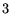xmatrix: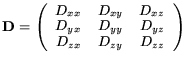(1)

The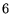independent values (the tensor is symmetric) of the tensor elements vary continuously with the spatial location in the tissue. Eigenvalues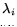and eigenvectors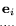of a matrix (1) can be found as a solution to the eigenvalue problem: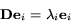(2)

Since the tensor is symmetric, its eigenvalues are always real numbers, and the eigenvectors are orthogonal and form a basis. Geometrically, a diffusion tensor can be thought of as an ellipsoid with its three axes oriented along these eigenvectors, with the three semi-axis lengths proportional to the square root of the eigenvalues of the tensor - mean diffusion distances [Basser et al. 1994]. Using the ellipsoidal interpretation, one can classify the diffusion properties of a tissue according to the shape of the ellipsoids, with extended ellipsoids corresponding to regions with strong linear diffusion (long, thin cells), flat ellipsoids to planar diffusion, and spherical ellipsoids to regions of isotropic media (such as fluid-filled regions like the ventricles). The quantitative classification can be done through the coefficients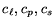[Westin et al. 1997] corresponding to linear, planar and spherical diffusion.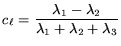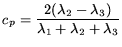(3)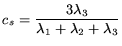These coefficients are normalized to the range of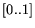. Values of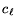that are close to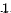selects regions with strong linear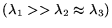diffusion. Values of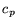and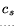will be small in those regions. Largevalues correspond to planar diffusion, largevalues corresponds to isotropic media. Due to the structure of the heart muscle, we will use the sum of theandcoefficients to characterize the tissue, thus looking for regions with high combined directional and planar anisotropies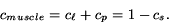(4)

This approach retains all of the directional information from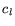andand discards all nondirectional.

Next: Algorithm Up: Method Previous: Method
Leonid Zhukov 2003-09-10